Bio macro molecules exercises (2010 VCE) 1) Enzymes are complex protein structures that function as biological catalysts. a. Why does a particular enzyme generally only catalyse a specific reaction? (1 mark) Solution Invertase is an enzyme which catalyses the conversion of sucrose to glucose and fructose. Invertase has a maximum activity temperature different from many other enzymes. The graph below shows the results of a study into the effects of both pH and temperature on the activity of invertase in sucrose solution.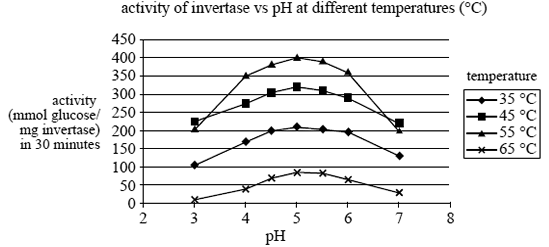b. At what temperature and pH does the enzyme in the study have maximum activity? Temperature __________ pH __________ (2 marks) Solution c. Why does changing the pH from the optimum value cause a decrease in the activity of the enzyme? (1 mark) Solution d. In this study the activity of the enzyme was measured as the number of millimole of glucose produced per milligram of enzyme (mmol glucose/mg invertase) in 30 minutes. Assuming excess sucrose, calculate the mass of glucose (Mr = 180) produced in 30 minutes from a sucrose solution containing 1.00 × 10–4 g of invertase if the measured activity is 300 mmol glucose/mg invertase. (2 marks) Solution2) The side chains of some amino acids are able to form amide (peptide) bonds. Glutathione is a tripeptide that protects cells in humans by acting as an antioxidant. The structure of glutathione is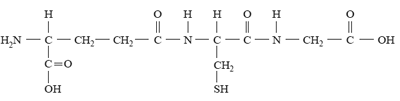The molecule of glutathione contains residues from the amino acids cysteine and glycine. Click for the data sheet information given on amino acids The name of the third amino acid found in glutathione is A. asparagine. B. aspartic acid. C. glutamine. D. glutamic acid. Solution 3) The following diagram is a simplified illustration of the protein insulin. Insulin consists of 51 amino acids arranged in two individual chains linked by disulfide bridges.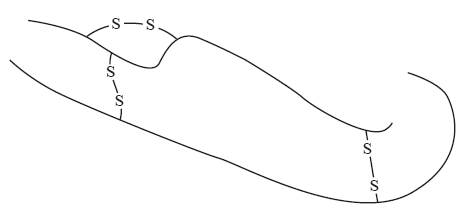How many peptide links are present in one molecule of insulin? A. 48 B. 49 C. 50 D. 51 Solution 4) Disulfide bridges are formed when the side chains of two amino acid residues react. The pair of amino acids that form the disulfide bridges could be A. cysteine and serine. B. cysteine and glycine. C. cysteine and cysteine. D. cysteine and glutamic acid. Solution5) The structure of Tamiflu®, an antiflu drug, is shown below.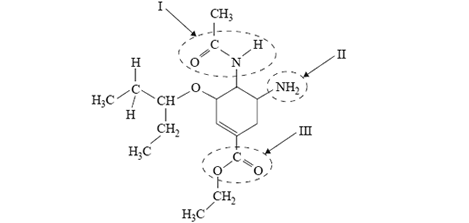The names of the functional groups labelled I, II and III are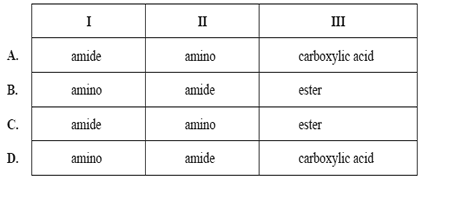Solution6) A single strand of DNA consists of a long chain of monomers called nucleotides. The structure of one of these nucleotides of DNA is shown below. Each nucleotide will polymerise with other nucleotides to form a single strand of DNA. Part of this nucleotide will also form bonds with a complementary nucleotide on a parallel strand of DNA forming the double helix structure.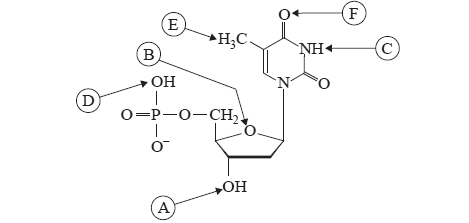a. Circle only the letters which represent the sites where this nucleotide can form bonds with other nucleotides to form a single strand of DNA. A B C D E F (2 marks) Solution b. i. Name the type of bonding that holds the two strands in human DNA together. Solution ii. Circle only the letters that represent the locations where these bonds between the two strands of DNA are formed. A B C D E F (1 + 2 = 3 marks) Solution